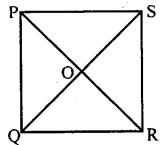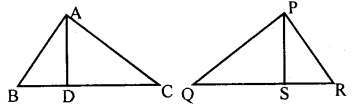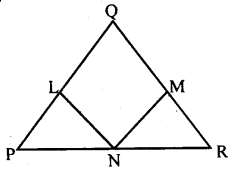# KSEEB Solutions for Class 8 Maths Chapter 11 Congruency of Triangles Ex 11.5

Students can Download Maths Chapter 11 Congruency of Triangles Ex 11.5 Questions and Answers, Notes Pdf, KSEEB Solutions for Class 8 Maths helps you to revise the complete Karnataka State Board Syllabus and score more marks in your examinations.

## Karnataka Board Class 8 Maths Chapter 11 Congruency of Triangles Ex 11.5

Question 1.
In ∆ ABC, AC = AB and the altitude AD bisects BC prove that ∆ ADC = ∆ ADB.In ∆ ABD and ∆ ACD
AB = AC [data]
BD = DC [data]
∴ ∆ ABD = ∆ ACD [SSS postulate].

Question 2.
In a square PQRS, diagonals bisect each other at O. Prove that: ∆ POQ = ∆ QOR = ∆ ROS = ∆ SOP∆ POQ and ∆ QOR
PQ = QR [Sides of a square]
PO = OR [data]
OQ = OQ [Common side]
∴ ∆ POQ = ∆ QOR [SSS postulate] …i In AQOR and AROS QR = RS [Sides of a square]
QO = OS [data]
OR = OR [Common side]
∴ ∆ QOR = ∆ ROS [SSS postulate] …ii
In ∆ROS and ∆SOP
SR = SP [Sides of a square]
RO = OP [data]
OS = OS [Common side]
∴ ∆ ROS ≅ ∆SOP [SSSpostulate]…iii
From (i), (ii) and (iii)
∆POQ ≅ ∆QOR ≅ ∆ROS ≅ ∆SOP

Question 3.
In figure two sides AB, BC, and the median AD of ∆ABC are respectively equal to two sides PQ, QR, and median PS of ∆PQR. Prove that
Does if follow that triangles ABC and PQR are congruent.Ans
1. IN ∆ ADB and ∆ PSQ
AB = PQ [data]
BD = QS [BC = QR, D and S are the mid points]
∆ ABD ≅ ∆PQR [SSS postulate]
∠APB = ∠ PSQ [Corresponding sides]

2. ∆ ADC and ∆ PSR
AB = PQ [data]
DC = SR
[BC = QR, D and S are the mid points]
∠PSR = 180 -∠PSQ
∆ ABC ≅ ∆ PSR [SAS postulate]
Yes from the results of (i) and( ii)
∆ ABC = ∆ PQR

Question 4.
In ∆ PQR, PQ = QR, L, M, and N are the midpoint of the sides of PQ, QR, and RP respectively prove that LN = MN